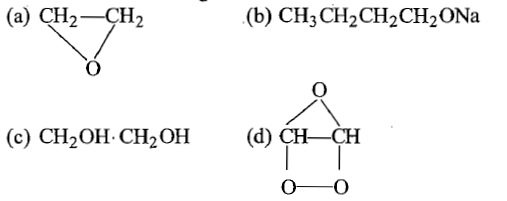1. 48% alcohol by mass

2. 10% alcohol by mass

3. 5% alcohol by mass

4. 90% alcohol by mass

Concept Questions :-

Alcohols: Preparation, Physical and Chemical Properties
High Yielding Test Series + Question Bank - NEET 2020

Difficulty Level:

The correct order of solubility of 1$°$,2$°$ and 3$°$ alcohol in water is:

(a) 3$°$>2$°$>1$°$                              (b) 1$°$>2$°$>3$°$

(c) 3$°$>1$°$>2$°$                              (d) none of these

Concept Questions :-

Alcohols: Preparation, Physical and Chemical Properties
High Yielding Test Series + Question Bank - NEET 2020

Difficulty Level:

Which of the following is an alkoxide?Concept Questions :-

Ethers: Preparation, Physical & Chemical Properties, Uses
High Yielding Test Series + Question Bank - NEET 2020

Difficulty Level:

The value of C-O-C angle in ether molecule is :

1. 180$°$

2. 150$°$

3. 90$°$

4. 110$°$

Concept Questions :-

Ethers: Preparation, Physical & Chemical Properties, Uses
High Yielding Test Series + Question Bank - NEET 2020

Difficulty Level:

Ethyl alcohol is also known as:

1. spirit of wine

2. methyl carbinol

3. grain alcohol

4. all of these

Concept Questions :-

Alcohols: Preparation, Physical and Chemical Properties
High Yielding Test Series + Question Bank - NEET 2020

Difficulty Level:

The products formed in the following reaction, C6H5-O-CH3 + HI $\stackrel{\mathrm{Heat}}{\to }$ are:

(a) C6H5OH and CH3I                 (b) C6H5I and CH3OH

(C) C6H5CH3 and HOI                 (d) C6H6 and CH3OI

Concept Questions :-

Phenols: Preparation, Properties, Acidic Nature of Phenol
High Yielding Test Series + Question Bank - NEET 2020

Difficulty Level:

The formula of phenoxy benzene is :

1. C6H5C6H5                             2. C6H5-O-C6H5

3. C6H5-O-C6H6                         4. none of these

Concept Questions :-

Ethers: Preparation, Physical & Chemical Properties, Uses
High Yielding Test Series + Question Bank - NEET 2020

Difficulty Level:

CH$\equiv$CH$\stackrel{{\mathrm{O}}_{3}/\mathrm{NAOH}}{\to }$X$\stackrel{\mathrm{Zn}/{\mathrm{CH}}_{3}\mathrm{COOH}}{\to }$Y is:

(a) CH2OH-CH2OH                   (b)CH3CH2OH

(c) CH3COOH                           (d)CH3OH

Concept Questions :-

Alcohols: Preparation, Physical and Chemical Properties
High Yielding Test Series + Question Bank - NEET 2020

Difficulty Level:

Which of the following reacts with water

(a) CHCl3                                     (b) CCl4

(c) CCl3CHO                                (d) CH2ClCH2Cl

Concept Questions :-

Alcohols: Preparation, Physical and Chemical Properties
High Yielding Test Series + Question Bank - NEET 2020

Difficulty Level:

Given are cyclohexanol (I), acetic acid (II), 2,4,6-trinitrophenol (III) and phenol (IV). In these, the order of decreasing acidic character will be

(a) III>II>IV>I                        (b) II>III>I>IV

(c) II>III>IV>I                        (d) III>IV>II>I

Concept Questions :-

Phenols: Preparation, Properties, Acidic Nature of Phenol